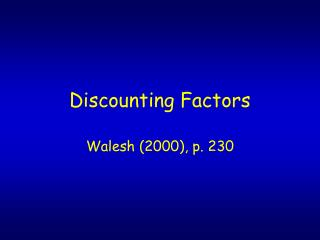DownloadDownload PresentationDiscounting Factors

# Discounting Factors

Télécharger la présentation## Discounting Factors

- - - - - - - - - - - - - - - - - - - - - - - - - - - E N D - - - - - - - - - - - - - - - - - - - - - - - - - - -
##### Presentation Transcript

1. Discounting Factors Walesh (2000), p. 230

2. Discounting factors are used to convert a set of discrete and continuous costs and revenues to a common point in time or to a common period.

3. Discounting factors account for the time value of money, and allow you to compare apples to apples.

4. Fig 8-6

5. Example 1 Given: \$10,000 is invested at 6% for four years. Earnings occur at simple interest with a single payment at the end of the four-year period. Find: Value of the single payment, that is, the original payment plus interest earned, at the end of four years. Solution: F = P(1+ni) = \$10,000(1 + 4(0.06)) = \$12,400

6. Total interest = ? \$2400 What is the difference between simple and compound interest? The investor does not earn interest on interest -- and the borrower does not pay interest.

7. Fig 8-7

8. Example 2 Given: \$10,000 is invested at 6% for four years. Interest is compounded annually and a single payment is to be made at the end of four years. Find: Value of the single payment, i.e., original principal plus total interest earned (interest on interest) at the end of 4 years. Solution: F = P(1+i)n = 10,000(1.06)4 = \$12,625

9. Total interest is \$2625, or \$225 more that the \$2400 interest earned from the simple interest version of the example. What if the interest is compounded monthly? i = 0.06/12 = 0.005 & n = 48 F = 10,000(1.005)48 = \$2705

10. Fig 8-8

11. Example 7 Given: As a result of a trust established for you, you will receive \$75,000 in ten years. Find: Present value of your trust on the assumption that interest is 6%. Solution: P = F/(1+i)n = \$75,000/ (1.06)10 = \$41,880

12. \$41,880 is the single-payment compound-amount that would need to be invested at 6% to yield \$75,000 in ten years.

13. The examples used up to now work with discrete (single) expenses, costs, incomes, and revenues. Another class of transactions is those involving a series of equal costs or incomes. Annuities are transactions involving a series of equal disbursements and/or receipts.

14. Fig 8-9

15. Fig 8-10

16. Example 8 Given: Starting this year, you decide to put \$2000 per year at the end of each year into a retirement plan earning 8%. Find: Value after 10 years and interest earned in 10 years. Solution: F = A((1+i)n – 1)/i = 2000(1.0810 – 1)/(0.08) = \$28,973 Future value = \$28,973, and interest = \$28972 – (10)2000 = \$8973.

17. Fig 8 –11

18. Example 9 Given: A small manufacturing plant projects that it will need \$1 million for construction of a plant expansion in 10 years and can invest funds at 6% interest. Find: The amount invested at the end of each of 10 years to fund the expansion. Solution: A = F (i)/((1+i)n – 1) = \$1,000,000(0.06)/(0.7908) = \$75,870

19. Example 10 (Series Present-Worth Example) Given: Annual O&M costs for a left-handed wrench painter are \$11,500 and the economic life is 20 years. Find: The present worth of the O&M costs with interest = 6%. Solution: P = A((1+i)n – 1)/((i)(1+i)n) = \$11500(1.0620 – 1)/(0.1924) = \$131,902 Why would you want to know this?

20. Fig 8-13

21. Example 11 Given: Your new car costs \$25,000. You pay \$2500 down and finance the remainder for 36 months at 10%. Find: Monthly payments and total interest paid. Solution: i = 0.10/12 = 0.00833 A = P((i)(1+i)n/((1+i)n – 1) = \$22500(0.00833)1.0083336/(1.0083336 – 1) =\$725.96 monthly payment Interest = (36)725.96 – 22500 = \$3634.56 Do you pay equal amounts of interest each month?

22. Example 12 Gradient-Series Present-Worth Given: For equipment being considered, the O&M cost is \$1100 for the first year, and the cost per year increases at \$100 per year. Find: Present worth of the O&M expenditures. Why would this be worth knowing? Can anyone give an example? Solution: Break the problem into an annuity and into a gradient series, as shown in Fig 8-15.

23. Fig 8-15

24. For the annual series (annuity) P = A(P/A, i, n) = 1000((1.0610 – 1)/((0.06)(1.06)10) P = \$7360 For the gradient series P = G(GSPWF) = \$3696 What is another way to work this problem?

25. Page 239, Example 13